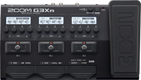# Meu efeito de solo

Discussion in 'Zoom G3n/G3Xn/G5n' started by RUDSON, Sep 27, 2018.

1. Meu efeito de soloDevice: Zoom G3Xn
Firmware: 2.00

Name on device: Slash Solo
Optimized for: Phones/Speaker

Effects chain:G3xn

Effect: "ZNR" (Dynamics), active - "yes"
"Detect" = GTRIN
"Depth" = 100
"Threshold" = 14
"Decay" = 0

Effect: "ParaEQ" (Filter), active - "yes"
"Frequency" = 125Hz
"Q" = 1.0
"Gain" = 4.0
"Volume" = 80

Effect: "MetalWRLD" (Overdrive / Distortion), active - "yes"
"Gain" = 80
"Bass" = 50
"Treble" = 50
"Volume" = 40

Effect: "Gt GEQ" (Filter), active - "yes"
"160 Hz" = 3.0
"400 Hz" = 2.0
"800 Hz" = -2.0
"3.2 kHz" = -1.0
"6.4 kHz" = 3.0
"12.0 kHz" = 0.0
"Volume" = 80
"160 Hz" = 0.0
"400 Hz" = 0.0
"800 Hz" = 0.0
"3.2 kHz" = 0.0
"6.4 kHz" = 0.0
"12.0 kHz" = 0.0
"Volume" = 80
"CH SEL" = LATCH
"Parameter set" = A

Effect: "Church" (Reverb), active - "yes"
"PreD" = 96
"Decay" = 49
"Mix" = 30
"Tail" = On

Effect: "TapeEcho" (Delay), active - "yes"
"Time" = 561
"F.B." = 64
"Mix" = 56
"Tail" = Off

Patch Volume: 100